# Excel COUNT and COUNTA functions to count cells

This short tutorial explains the basics of the Excel COUNT and COUNTA functions and shows a few examples of using a count formula in Excel. You will also learn how to use the COUNTIF and COUNTIFS functions to count cells that meet one or more criteria.

As everyone knows, Excel is all about storing and crunching numbers. However, apart from calculating values, you may also need to count cells with values - with any value, or with specific value types. For example, you may want a quick count of all items in a list, or the total of inventory numbers in a selected range.

Microsoft Excel provides a couple of special functions for counting cells: COUNT and COUNTA. Both all very straightforward and easy-to-use. So let's take a quick look at these essential functions first, and then I will show you a few Excel formulas to count cells that meet certain condition(s), and clue you in on the quirks in counting some value types.

## Excel COUNT function - count cells with numbers

You use the COUNT function in Excel to count the number of cells that contain numerical values.

The syntax of the Excel COUNT function is as follows:

COUNT(value1, [value2], …)

Where value1, value2, etc. are cell references or ranges within which you want to count cells with numbers.

In the modern versions of Excel 2016, Excel 2013, Excel 2010, and Excel 2007, the COUNT function accepts up to 255 arguments. In earlier Excel versions, you can supply up to 30 'values'.

For example, the following formula returns the total number of numeric cells in range A1:A100:

`=COUNT(A1:A100)`

Note. In the internal Excel system, dates are stored as serial numbers and therefore the Excel COUNT function counts dates and times as well.

### Using COUNT function in Excel - things to remember

Below are the two simple rules by which the Excel COUNT function works.

1. If an argument(s) of an Excel Count formula is a cell reference or range, only numbers, dates and times are counted. Blanks cells and cells containing anything but a numeric value are ignored.
2. If you type values directly into the Excel COUNT arguments, the following values are counted: numbers, dates, times, Boolean values of TRUE and FALSE, and text representation of numbers (i.e. a number enclosed in quotation marks like "5").

For example, the following COUNT formula returns 4, because the following values are counted: 1, "2", 1/1/2016, and TRUE.

`=COUNT(1, "apples", "2", 1/1/2016, TRUE)`

### Excel COUNT formula examples

And here are a few more examples of using the COUNT function in Excel on different values.

To count cells with numeric values in one range, use a simple count formula like

`=COUNT(A2:A10)`

The following screenshot demonstrates which types of data are counted and which are ignored:To count several non-contiguous ranges, supply all of them to your Excel COUNT formula. For example, to count cells with numbers in columns B and D, you can use formula similar to this:

`=COUNT(B2:B7, D2:D7)`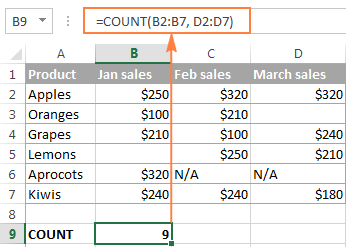Tips:

• If you want to count numbers that meet certain criteria, use either the COUNTIF or COUNTIFS function.
• If apart from numbers, you also want to count cells with text, logical values and errors, use the COUNTA function, which leads us right to the next section of this tutorial.

## Excel COUNTA function - count cells with values (non-blank cells)

The COUNTA function in Excel counts the number of cells in a range that are not empty.

The syntax of the Excel COUNTA function is akin to that of COUNT:

COUNTA(value1, [value2], …)

Where value1, value2, etc. are cell references or ranges where you want to count non-blank cells.

For example, to count cells with value in range A1:A100, use the following formula:

`=COUNTA(A1:A100)`

To count non-empty cells in several non-adjacent ranges, use a COUNTA formula similar to this:

`=COUNTA(B2:B10, D2:D20, E2:F10)`

As you can see, the ranges supplied to an Excel COUNTA formula do not necessarily need to be of the same size, i.e. each range may contain a different number of rows and columns.

Please keep in mind that Excel's COUNTA function counts cells containing any type of data, including:

• Numbers
• Dates / times
• Text values
• Boolean values of TRUE and FALSE
• Error values like #VALUE or #N/A
• Empty text strings ("")

In some cases, you may be perplexed by the COUNTA function's result because it differs from what you see with your own eyes. The point is that an Excel COUNTA formula may count cells that visually look empty, but technically they are not. For example, if you accidentally type a space in a cell, that cell will be counted. Or, if a cell contains some formula that returns an empty string, that cell will be counted as well.

In other words, the only cells that the COUNTA function does not count are absolutely empty cells.

The following screenshot demonstrates the difference between Excel COUNT and COUNTA functions: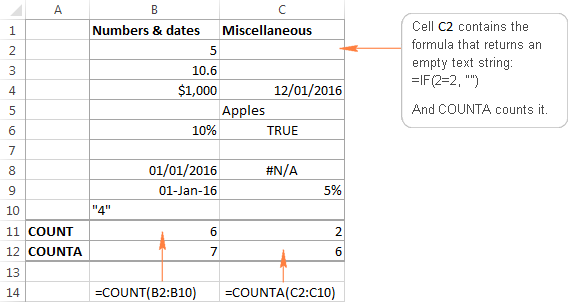Tip. If you just want a quick count of non-blank cells in a selected range, simply have a look at Status Bar at the bottom right corner of your Excel window: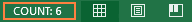## Excel COUNTIF function - count cells that meet one condition

The COUNTIF function is purposed for counting cells that meet a certain criterion. Its syntax requires 2 arguments, which are self-explanatory:

COUNTIF(range, criteria)

In the first argument, you define a range where you want to count cells. And in the second parameter, you specify a condition that should be met.

For example, to count how many cells in range A2:A15 are "Apples", you use the following COUNTIF formula:

`=COUNTIF(A2:A15, "apples")`

Instead if typing a criterion directly in the formula, you can input a cell reference as demonstrated in the following screenshot: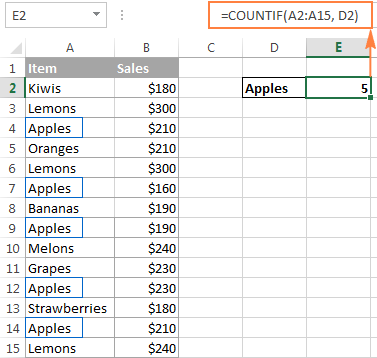For more information about using the COUNTIF function in Excel, check out the following tutorial:
COUNTIF in Excel - count if not blank, greater than, duplicate or unique

## Excel COUNTIFS function - count cells that match several criteria

The COUNTIFS function is similar to COUNTIF, but it allows specifying multiple ranges and multiple criteria. Its syntax is as follows:

COUNTIFS(criteria_range1, criteria1, [criteria_range2, criteria2]…)

The COUNTIFS function was introduced in Excel 2007 and is available in all later versions of Excel 2010, 2013, and 2016.

For example, to count how many "Apples" (column A) have made \$200 and more sales (column B), you use the following COUNTIFS formula:

`=COUNTIFS(A2:A15,"apples", B2:B15,">=200")`

And again, to make your COUNTIFS formula more versatile, you can supply cell references as the criteria: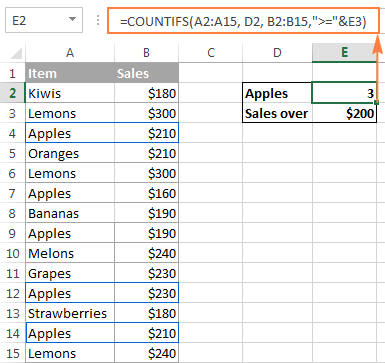You will find plenty more formula examples here: How to use Excel COUNTIFS function with multiple criteria.

## Count the number of cells in a range (ROWS and COLUMNS functions)

If you need to find out the total number of cells in a rectangular range, utilize the ROWS and COLUMNS functions, which return the number of rows and columns in an array, respectively:

`=ROWS(range)*COLUMNS(range)`

For example, to find out how many cells there are in a given range, say A1:D7, use the following formula:

`=ROWS(A1:D7)*COLUMNS(A1:D7)`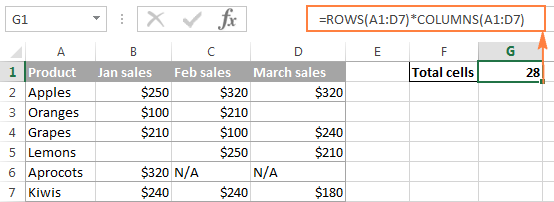Well, this is how you use the Excel COUNT and COUNTA functions. Like I said, they are very straightforward and you are unlikely to run into any difficulty when using your count formula in Excel. If someone knows and is willing to share some interesting tips on to how to count cells in Excel, your comments will be greatly appreciated. I thank you for reading and hope to see you on our blog next week!